Generalized symmetries of an 𝓝 = 1 supersymmetric Boiti–Leon–Manna–Pempinelli system*
Wang Jian-Yonga),b), Tang Xiao-Yanc),d)†, Liang Zu-Fenge), Lou Sen-Yuef),g)
Department of Mathematics and Physics, Quzhou University, Quzhou 324000, China
Department of Physics and Astronomy, Shanghai Jiao Tong University, Shanghai 200240, China
Institute of Systems Science, East China Normal University, Shanghai 200241, China
The Abdus Salam International Center for Theoretical Physics, Trieste 34100, Italy
Department of Physics, Hangzhou Normal University, Hangzhou 310036, China
Ningbo Collabrative Innovation Center of Nonlinear Harzard System of Ocean and Atmosphere, Ningbo University, Ningbo 315211, China
Shanghai Key Laboratory of Trustworthy Computing, East China Normal University, Shanghai 200062, China

Corresponding author. E-mail: xytang@sist.ecnu.edu.cn

*Project supported by the National Natural Science Foundation of China (Grant Nos. 11275123, 11175092, 11475052, and 11435005), the Shanghai Knowledge Service Platform for Trustworthy Internet of Things, China (Grant No. ZF1213), and the Talent Fund and K CWong Magna Fund in Ningbo University, China.

Abstract

The formal series symmetry approach (FSSA), a quite powerful and straightforward method to establish infinitely many generalized symmetries of classical integrable systems, has been successfully extended in the supersymmetric framework to explore series of infinitely many generalized symmetries for supersymmetric systems. Taking the 𝒩 = 1 supersymmetric Boiti–Leon–Manna–Pempinelli system as a concrete example, it is shown that the application of the extended FSSA to this supersymmetric system leads to a set of infinitely many generalized symmetries with an arbitrary function f ( t). Some interesting special cases of symmetry algebras are presented, including a limit case f ( t) = 1 related to the commutativity of higher order generalized symmetries.

Keyword: 02.30.Jr; 11.30.Pb; 02.20.Sv; formal series symmetry approach; generalized symmetry; infinite dimensional Lie algebra; supersymmetric Boiti–Leon–Manna–Pempinelli system
1. Introduction

Symmetry is an essential issue for nonlinear differential equations. However, compared with classical integrable systems, there is quite limited progress on symmetries of supersymmetric systems. As is known, many effective methods have been established to study various symmetries of classical nonlinear differential systems, for instance, the famous Lie group approaches[1, 2] and the direct method have been developed to study Lie point symmetries of partial differential equations. These methods can also be utilized to study symmetries of the SUSY equations. Recently, the classical Lie group approach has been used to find Lie symmetries, symmetry reductions and similarity solutions of the (2+ 1)-dimensional supersymmetric Boussinesq equation. This approach has also been applied to the coupled bosonic system of the supersymmetric system obtained by the bosonization approach through introducing some fermionic parameters in the expansion of the superfield. In addition, the nonlocal symmetry related to the Bä cklund transformation, also called residual symmetry, has been studied for the supersymmetric KdV equation and related interesting interacting wave solutions have been found. Earlier symmetry related studies include the discrete symmetries of the 𝒩 = 2 supersymmetric modified nonlinear Schrö dingier (NLS) hierarchy and the 𝒩 = 2 supersymmetric generalized NLS hierarchies,  the hidden symmetries of the 𝒩 = 4 supersymmetric Yang– Mills equations,  and the additional (non-isospectral) symmetries of the supersymmetric integrable hierarchies of Kadomtsev– Petviashvili type models.

The formal series symmetry approach (FSSA) is famous for finding infinitely many generalized symmetries of integrable systems.[13, 14] It has been revealed that many famous (2+ 1)-dimensional physical models, such as the Kadomtsev– Petviashvili equation,  the Toda field equation,  dispersive long wave equations,  the Burgers system,  etc., admit one or more sequences of infinitely many generalized symmetries obtained by means of the FSSA. In this paper, taking an 𝒩 = 1 supersymmetric Boiti– Leon– Manna– Pempinelli system as an illustration, we for the first time intend to demonstrate that the FSSA can be extended in the supersymmetric framework to explore infinitely many generalized symmetries of supersymmetric systems.

The 𝒩 = 1 supersymmetric Boiti– Leon– Manna– Pempinelli (SUSY BLMP) system was given in a recent paper written by Delisle and Mosaddeghi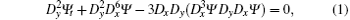where Di (i = x, y) denotes the supercovariant derivative defined as Di = θ + θ i. It is an 𝒩 = 1 extension of the classical BLMP equation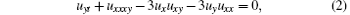obtained as usual by extending the space (x, y, t) to the superspace (x, y, t; θ ), where θ is a Grassmann variable satisfying θ 2 = 0. In addition, the bilinear form, multisolitons and bilinear Bä cklund transforms for the SUSY BLMP equation have been obtained. It has also been pointed out that the BLMP equation is strongly related to the Korteweg-de Vries (KdV) equation, and naturally, the SUSY BLMP can be reduced to the SUSY KdV equation. The 𝒩 = 1 SUSY extension of the KdV equation was first formulated by Manin and Radul. Similar to the role of the classical KdV equation in two-dimensional quantum gravity, [20, 21] it has been indicated that the 𝒩 = 1 SUSY extensions of the KdV equation might be relevant in the study of SUSY 2D quantum gravity.[22, 23] Such a significant role of the SUSY KdV equation has prompted ongoing theoretical investigations and a number of interesting properties have been established, for instance the bi-Hamiltonian structures,  the Painlevé property,  the Darboux transformation,  the Bä cklund transformation and bilinear forms.[28, 29]

To apply the FSSA to the SUSY BLMP equation  (1), let us first take the following finite Taylor expansion: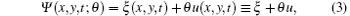where ξ is a fermonic (odd) function and u is a bosonic (even) function, to transform the SUSY BLMP equation  (1) into a system of two equations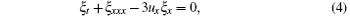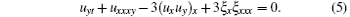It is evident that in the fermionic limit (ξ = 0), the above system is reduced to the classical BLMP equation  (2). In Section 2, a set of infinitely many generalized symmetries of the SUSY BLMP system of Eqs.  (4) and (5) is obtained. The related symmetry algebras in some interesting special cases are presented in Section  3. The last section is devoted to the summary and discussion.

2. Infinitely many generalized symmetries

A symmetry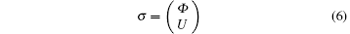of the SUSY BLMP system is defined as a solution of its linearized system by assuming that equations  (4) and (5) are form invariant under the following infinitesimal transformation: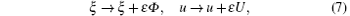where ɛ is an infinitesimal parameter, Φ is a fermionic function, and U is a bosonic function. Substituting the infinitesimal transformation  (7) into Eqs.  (4) and (5) and picking up coefficients of ɛ arrive at the linearized equations that symmetries Φ and U should satisfy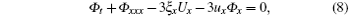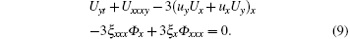Applying the formal series symmetry theory[13, 14] in the SUSY framework, we can expand symmetries Φ and U in the form of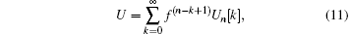respectively, where the fermionic function Φ n[k] and the bosonic function Un[k] are functions of x, y, u, ξ and their arbitrary derivatives, but not t dependent explicitly, f is an arbitrary function of t, and f(nk) denotes the (nk)-th derivative of f with respect to t.

The substitution of Eqs.  (10) and (11) into Eqs.  (8) and (9) leads to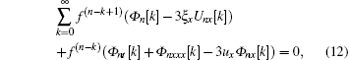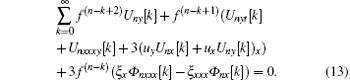Keeping the function f arbitrary requires the coefficients of different derivatives of f in the above two equations vanish identically. Therefore, by setting to zero all the coefficients of different derivatives of f, we obtain the following overdetermined equations: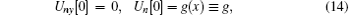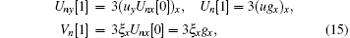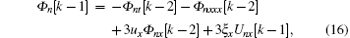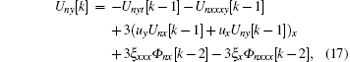for k = 2, 3, … , n + 1, and we have defined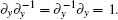.

It is noted that Un[k − 1] and Vn[k] can be solved recursively from Eqs.  (14)– (17) for a given g. The results can be expressed in the matrix form as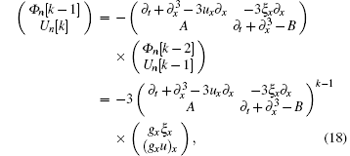for k = 2, 3, … , n + 1, where the operators A and B are given by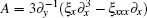and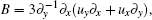, respectively.

Similar to the classical case, the truncate conditions should be introduced so that meaningful generalized symmetries can be obtained, which are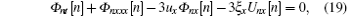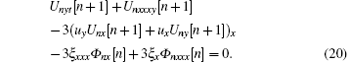Equivalently, Φ = Φ n[n] and U = Un[n + 1] satisfy the symmetry definition equations  (8) and (9). Then in order to deduce explicit generalized symmetries, one has to fix an exact expression of the x-dependent function g in Eq.  (18). Similarly, with the help of the dimensional analysis, one can find that the only possible selection of g is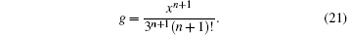Consequently, with this particular g given in Eq.  (21), explicit generalized symmetries can be produced for the SUSY BLMP system of Eqs.  (4) and (5). In principle, one can derive infinitely many generalized symmetries explicitly from the recursion equation, however, due to the difficulty in determining the integration functions, it is troublesome to write down explicit generalized symmetries for n > 2. Therefore, here we just present the first three explicit generalized symmetries, obtained by taking g = x/3,   x2/18, and x3/162, respectively,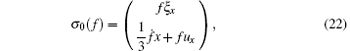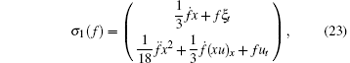and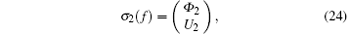with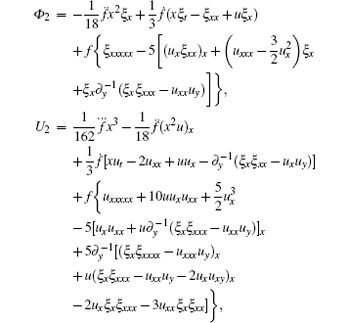where the dot over the function denotes the derivative of the function with respect to t.

It is remarkable that in the derivation of symmetries  (22)– (24), one can utilize the following tips to avoid the complicated computation caused by the anti-commuting fermionic functions. First, restrict the lower-order x-derivative of ξ placed in front of its higher orders, for instance, the term like ξ xxxξ x should be rewritten as − ξ xξ xxx. Second, to prove that the first one of the positive flow (taking f = 1 in σ 2(f)) also satisfies the symmetry definition equations  (8) and (9), it is convenient to use the substitutions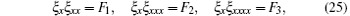where F1, F2, and F3 are all bosonic functions. Thus, σ 2(1) can be reformed as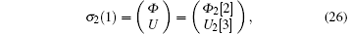with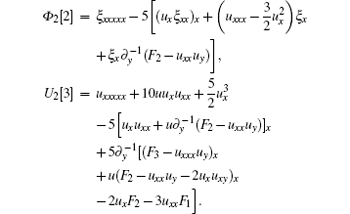With the substitution  (25), equation  (5) becomes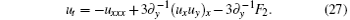Hereafter, we mainly need to consider the anti-commuting relations involving the x-derivatives of the bosonic functions F1, F2, and F3, while other anti-commuting relations can be readily verified, when equations  (26) and (27) are inserted into the symmetry definition equations  (8) and  (9).

3. Symmetry algebra

The symmetries of the SUSY BLMP system can be given in a compact form by substituting Eq.  (21) into Eqs.  (10) and (11), that is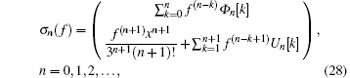with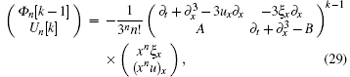for k = 1, 2, … , n+ 1.

It is interesting to observe that the presence of the arbitrary function f in the generalized symmetries leads to a closed infinite-dimensional Lie algebra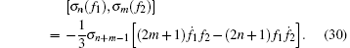Actually, it is not surprising that σ n(f) constitutes the algebra  (30), because the symmetries of the classical BLMP equation constitute the same algebra. We also note that this algebra is isomorphic to those of the (2+ 1)-dimensional modified KdV type systems.[30, 31] Three interesting subalgebras of  Eq.  (30) are listed as follows.

Case 1f1 = f2 = 1. In this particular case, generalized symmetries with the presence of an arbitrary function f(t) degrade to t-independent symmetries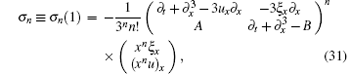which constitute a commuting algebra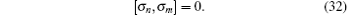Such algebra implies the commutativity of the positive flow.

Case 2f = t. In this case, σ n(t) = τ n, called τ symmetries, linearly depend on the variables x, y, and t. The set of symmetries constitutes a centerless Virasoro algebra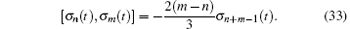It is well known that the Virasoro algebra of the asymptotic symmetry plays an important role in understanding the quantum nature of black holes.

Case 3f = tr, and r ≠ 1 is an integer. In this case, we find that the general algebra reduces to w type algebra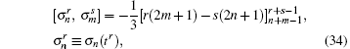which contains all the conformal spins and plays a crucial role in some areas of physics, such as the sl(∞ ) Toda theory, [14, 33] integrable systems, [13, 34] membrane theory, W string and W gravity theories.

4. Summary and discussion

In this paper, we have demonstrated that the 𝒩 = 1 SUSY BLMP equation admits a set of infinitely many generalized symmetries with an arbitrary function f(t). It has also been shown that these symmetries constitute a closed infinite-dimensional Lie algebra which is a generalization of the w type algebra. Thus we conclude that the SUSY BLMP equation is integrable in the sense of symmetry.

In addition, one can obtain in a similar way that the SUSY BLMP equation also admits a special simple symmetry with an arbitrary function p = p(y),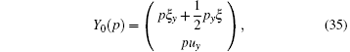which is commuting with the generalized symmetries σ n(f) by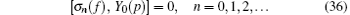Furthermore, from Eqs.  (22), (23), and (35), one can find the SUSY BLMP possesses the following infinite-dimensional Lie point symmetries of the form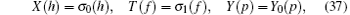where f and h are arbitrary functions of t, and p is an arbitrary function of y. In such a special selection, it is effortless to check that the nonzero commutation relations of these symmetries are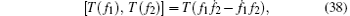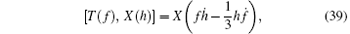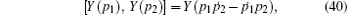which constitute an infinite-dimensional Kac– Moody– Virasoro– type algebra.

The additional properties of the SUSY BLMP equation, and what we know about the SUSY extensions of (2+ 1)-dimensional classical integrable systems are worthy of further study. As mentioned above, it has been confirmed that the equations of motions and symmetries in the quantum 2D gravity and the noncritical string theory at least c < 1 can be formulated in the KdV type equations.[20, 21] Similarly, it has also been indicated that the 𝒩 = 1 supersymmetric extensions of the KdV equation might be relevant in the study of the supersymmetric 2D quantum gravity.[22, 23] In the viewpoint of physics, the (2+ 1)-dimensional field theory is particularly interesting since the theory is simple yet possesses a rich asymptotic symmetry and provides us with a deeper insight in general. Consequently, it is significant to study supersymmetric extensions of the KdV equation, especially those in 2+ 1 dimensions.

Reference
 1 Olver P J 1993 Applications of Lie Groups to Differential Equations New York Springer [Cited within:1] 2 Bluman G W and Kumei S 1989 Symmetries and Differential Equations Berlin Springer [Cited within:1] 3 Clarkson P A and Kruskal M D 1989 J. Math. Phys. 30 2201 DOI:10.1063/1.528613 [Cited within:1] [JCR: 1.296] 4 Lou S Y 1990 Phys. Lett. A 151 133 DOI:10.1016/0375-9601(90)90178-Q [Cited within:1] [JCR: 1.11] 5 Lou S Y, Tang X Y and Lin J 2000 J. Math. Phys. 41 8286 DOI:10.1063/1.1320859 [Cited within:1] 6 Wang Y F, Lou S Y and Qian X M 2010 Chin. Phys. B 19 050202 DOI:10.1088/1674-1056/19/5/050202 [Cited within:1] [JCR: 1.148] [CJCR: 1.2429] 7 Gao X N, Yang X D and Lou S Y 2012 Commun. Theor. Phys. 58 617 DOI:10.1088/0253-6102/58/5/01 [Cited within:1] 8 Gao X N, Lou S Y and Tang X Y 2013 J. High Energy Phys. 05 029 DOI:10.1007/JHEP05(2013)029 [Cited within:1] [JCR: 5.618] 9 Sarin A 1997 Phys. Lett. B 395 218 DOI:10.1016/S0370-2693(97)00061-0 [Cited within:1] [JCR: 0.479] 10 Sorin A S 1998 Phys. Atom. Nucl. 61 1768 [Cited within:1] [JCR: 0.539] 11 Alexand er D P and Martin W 2007 Commun. Math. Phys. 275 685 DOI:10.1007/s00220-007-0296-1 [Cited within:1] [JCR: 1.971] 12 Nissimov S and Pacheva S 2002 J. Math. Phys. 43 2547 DOI:10.1063/1.1466533 [Cited within:1] [JCR: 1.296] 13 Lou S Y 1993 J. Phys. A: Math. Gen. 26 4387 DOI:10.1088/0305-4470/26/17/043 [Cited within:4] [JCR: 1.577] 14 Lou S Y 1993 Phys. Rev. Lett. 71 4099 DOI:10.1103/PhysRevLett.71.4099 [Cited within:4] [JCR: 7.943] 15 Lou S Y 1994 J. Phys. A: Math. Gen. 27 3235 DOI:10.1088/0305-4470/27/9/033 [Cited within:1] [JCR: 1.577] 16 Wang J Y, Liang Z F and Tang X Y 2014 Phys. Scr. 89 025201 DOI:10.1088/0031-8949/89/02/025201 [Cited within:1] 17 Delisle L and Mosaddeghi M 2013 J. Phys. A: Math. Theor. 46 115203 DOI:10.1088/1751-8113/46/11/115203 [Cited within:2] [JCR: 1.766] 18 Boiti M, Leon J J P, Manna M and Pempinelli F 1986 Inverse Probl. 2 271 DOI:10.1088/0266-5611/2/3/005 [Cited within:1] [JCR: 1.896] 19 Manin Y I and Radul A O 1985 Commun. Math. Phys. 98 65 DOI:10.1007/BF01211044 [Cited within:1] [JCR: 1.971] 20 Ghosh S and Paul S K 1995 Phys. Lett. B 341 293 DOI:10.1016/0370-2693(95)80007-K [Cited within:2] [JCR: 0.479] 21 Ghosh S and Sarma D 2001 Nuclear Phys. B 616 549 DOI:10.1016/S0550-3213(01)00434-5 [Cited within:2] [JCR: 4.327] 22 Alvarez-Gaumé L, Itoyama H, Mañnes J L and Zadra A 1992 Int. J. Mod. Phys. A 7 5337 DOI:10.1142/S0217751X92002441 [Cited within:2] [JCR: 1.127] 23 Alvarez-Gaumé L, Becker K, Becker M, Emparan R and Mañnes J L 1993 Int. J. Mod. Phys. A 8 2297 DOI:10.1142/S0217751X93000916 [Cited within:2] [JCR: 1.127] 24 Oevel W and Popowicz Z 1991 Commun. Math. Phys. 139 441 DOI:10.1007/BF02101874 [Cited within:1] [JCR: 1.971] 25 Mathieu P 1988 Phys. Lett. A 128 169 DOI:10.1016/0375-9601(88)90903-6 [Cited within:1] [JCR: 1.11] 26 Liu Q P 1995 Lett. Math. Phys. 35 115 DOI:10.1007/BF00750761 [Cited within:1] 27 Liu Q P and Xie Y F 2004 Phys. Lett. A 325 139 DOI:10.1016/j.physleta.2004.03.047 [Cited within:1] [JCR: 1.11] 28 Carstea A S 2000 Nonlinearity 13 1645 DOI:10.1088/0951-7715/13/5/312 [Cited within:1] [JCR: 1.602] 29 Carstea A S, Ramani A and Grammaticos B 2001 Nonlinearity 14 1419 DOI:10.1088/0951-7715/14/5/325 [Cited within:1] [JCR: 1.602] 30 Wang J Y, Yu J and Lou S Y 2010 Commun. Theor. Phys. 53 999 DOI:10.1088/0253-6102/53/6/01 [Cited within:1] 31 Wang J Y, Hu H W and Yu J 2011 J. Math. Phys. 52 103704 DOI:10.1063/1.3646091 [Cited within:1] [JCR: 1.296] 32 Strominger A 1998 J. High Energy Phys. 02 009 [Cited within:1] [JCR: 5.618] 33 Avan J 1992 Phys. Lett. A 168 363 DOI:10.1016/0375-9601(92)90519-R [Cited within:1] [JCR: 1.11] 34 Bakas I and Kiritsis E 1990 Nucl. Phys. B 343 185 DOI:10.1016/0550-3213(90)90600-I [Cited within:1] [JCR: 4.327] 35 Hoppe J 1982 Quantum Theory of a Massless Relativistic Surface and a Two-dimensional Bound State Problem(Ph. D. Thesis) (MIT) [Cited within:1] 36 Henneaux M and Rey S J 2010 J. High Energy Phys. 12 007 DOI:10.1007/JHEP12(2010)007 [Cited within:1] [JCR: 5.618]